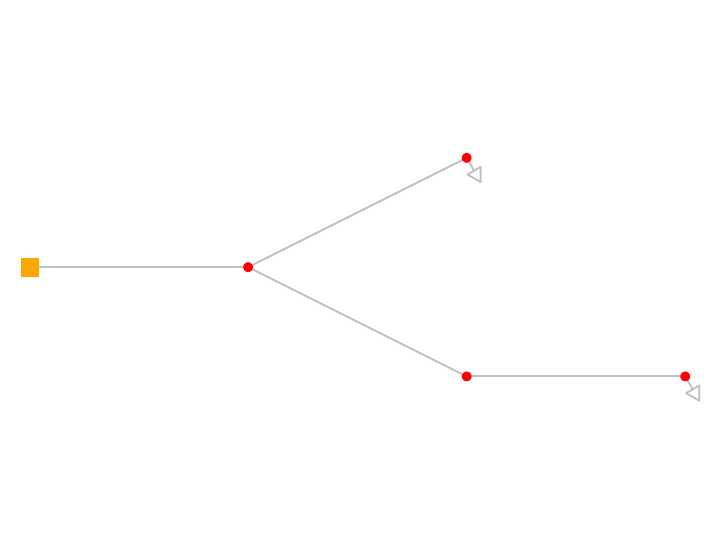# Creating a pandapipes network including height differences¶

This tutorial shows how to define junctions on different height levels. The following network will be created.### Empty network¶

At first, pandapipes is imported. We will then create an empty network container and specify the fluid. The fluid water from the pandapipes library is used.

In :
import pandapipes as pp

In :
net = pp.create_empty_network(fluid="water") # create an empty network


The create functions are used to create more components. The components will be added to the corresponding component tables.

### Junctions¶

In :
junction1 = pp.create_junction(net, pn_bar=1.0, tfluid_k=293.15, height_m = 352, name="Junction 1")
junction2 = pp.create_junction(net, pn_bar=1.0, tfluid_k=293.15, height_m = 358, name="Junction 2")
junction3 = pp.create_junction(net, pn_bar=1.0, tfluid_k=293.15, height_m = 361, name="Junction 3")
junction4 = pp.create_junction(net, pn_bar=1.0, tfluid_k=293.15, height_m = 346, name="Junction 4")
junction5 = pp.create_junction(net, pn_bar=1.0, tfluid_k=293.15, height_m = 400, name="Junction 5")


The junctions are now stored in the net and can be called. Some parameters, which were not explicitly mentioned in the last section, were defined using default values. Note that the parameters of the junctions above contain the parameter height_m, which defines the height above sea level of the junction. The stored potential energy is automatically respected by pandapipes.

In :
net.junction # show junction table

Out:
name pn_bar tfluid_k height_m in_service type
0 Junction 1 1.0 293.15 352.0 True junction
1 Junction 2 1.0 293.15 358.0 True junction
2 Junction 3 1.0 293.15 361.0 True junction
3 Junction 4 1.0 293.15 346.0 True junction
4 Junction 5 1.0 293.15 400.0 True junction

In the next steps, more components are added.

### External Grid¶

In the example, the external grid is connected to junction5. The pressure is at 0.5 bar.

In :
medium_pressure_grid = pp.create_ext_grid(net, junction=junction5, p_bar=0.5, t_k=293.15, name="Grid Connection")

net.ext_grid # show external grid table

Out:
name junction p_bar t_k in_service type
0 Grid Connection 4 0.5 293.15 True pt

### Pipes¶

The network contains four pipes. The lenghts and the junctions are displayed on the network figure. Pipe 4 is defined using a roughness k_mm of 0.5 mm, which deviates from the default value of 1 mm.

In :
pipe1 = pp.create_pipe_from_parameters(net, from_junction=junction1, to_junction=junction2, length_km=0.545, diameter_m=0.2,  name="Pipe 1")
pipe2 = pp.create_pipe_from_parameters(net, from_junction=junction2, to_junction=junction3, length_km=0.095, diameter_m=0.15, name="Pipe 2")
pipe3 = pp.create_pipe_from_parameters(net, from_junction=junction1, to_junction=junction4, length_km=0.285, diameter_m=0.15, name="Pipe 3")
pipe4 = pp.create_pipe_from_parameters(net, from_junction=junction1, to_junction=junction5, length_km=0.43, diameter_m=0.15, k_mm=0.5, name="Pipe 4")


Up to now, the pipe components table looks like the table displayed in the following section:

In :
net.pipe # show pipe table

Out:
name from_junction to_junction std_type length_km diameter_m k_mm loss_coefficient alpha_w_per_m2k text_k qext_w sections in_service type
0 Pipe 1 0 1 None 0.545 0.20 1.0 0.0 0.0 293.0 0.0 1 True pipe
1 Pipe 2 1 2 None 0.095 0.15 1.0 0.0 0.0 293.0 0.0 1 True pipe
2 Pipe 3 0 3 None 0.285 0.15 1.0 0.0 0.0 293.0 0.0 1 True pipe
3 Pipe 4 0 4 None 0.430 0.15 0.5 0.0 0.0 293.0 0.0 1 True pipe

The parameters from_junction and to_junction define the orientation of the pipe. If the flow enters the pipe at the from_junction node and leaves it through the to_junction node, the velocity will have a positive sign. Otherwise, it has a negative sign.

### Sinks¶

With a sink, a constant mass flow can be defined. Here, we will create two sinks with mass flows of 277 g/s and 139 g/s. Sinks and sources are always assigned to a specific junction. The sign of the mass flow is positive, if a mass flow is removed from the system.

In :
sink = pp.create_sink(net, junction=junction4, mdot_kg_per_s=0.277, name="Sink 1")
sink = pp.create_sink(net, junction=junction3, mdot_kg_per_s=0.139, name="Sink 2")
net.sink

Out:
name junction mdot_kg_per_s scaling in_service type
0 Sink 1 3 0.277 1.0 True sink
1 Sink 2 2 0.139 1.0 True sink

The calculation is now started. The pipe friction can be respected using different models. By default, the Nikuradse model is used. A distinction between laminar and turbulent flow is not implemented in pandapipes. Typically, pipe calculation software detects the kind of flow. However, for pandapipes applications, we usually can expect turbulent flow conditions.

Nevertheless, a distinction between laminar and turbulent flow will be added in the future.

In :
pp.pipeflow(net, friction_model="nikuradse")


The results are ready now and can be displayed with the following commands:

In :
net.res_junction  # calculated pressure and temperature at junctions

Out:
p_bar t_k
0 5.194289 293.15
1 4.607432 293.15
2 4.314000 293.15
3 5.780977 293.15
4 0.500000 293.15
In :
net.res_pipe  # velocities, mass flows through pipes and other results

Out:
v_mean_m_per_s p_from_bar p_to_bar t_from_k t_to_k mdot_from_kg_per_s mdot_to_kg_per_s vdot_norm_m3_per_s reynolds lambda
0 0.004433 5.194289 4.607432 293.15 293.15 0.139 -0.139 0.000139 886.106589 0.102569
1 0.007880 4.607432 4.314000 293.15 293.15 0.139 -0.139 0.000139 1181.475451 0.087337
2 0.015704 5.194289 5.780977 293.15 293.15 0.277 -0.277 0.000278 2354.451079 0.060350
3 -0.023584 5.194289 0.500000 293.15 293.15 -0.416 0.416 -0.000417 3535.926531 0.045036

## Plotting¶

Using matplotlib, the network can easily be plotted.

In :
import matplotlib
from pandapipes.plotting.simple_plot import simple_plot as sp

sp(net, plot_sinks = True)

hp.pandapipes.plotting.simple_plot - WARNING: No or insufficient geodata available --> Creating artificial coordinates. This may take some timeOut:
<AxesSubplot:>

As long as no coordinates have been explicitly added to the junctions, automatically generated coordinates are used.